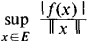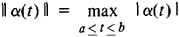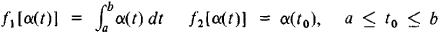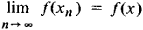# Linear Functional

Also found in: Wikipedia.

## linear functional

[′lin·ē·ər ′fəŋk·shən·əl]
(mathematics)
A linear transformation from a vector space to its scalar field.
McGraw-Hill Dictionary of Scientific & Technical Terms, 6E, Copyright © 2003 by The McGraw-Hill Companies, Inc.
The following article is from The Great Soviet Encyclopedia (1979). It might be outdated or ideologically biased.

## Linear Functional

a generalization of the concept of linear form to vector spaces. A number-valued function f defined on a normed vector space E is called a linear functional on E if

(1) f(x) is linear, that is,

f(αx + βγ) = αf(x) + βf(y)

where x and y are any element of E and a and β are numbers, and

(2) f(x) is continuous. The continuity of f is equivalent to the requirement that ǀf(x)ǀ/ǀǀxǀǀ be bounded on E; in the latter case, the quantityis called the norm of f and designated by ǀǀfǀǀ. Let C[a, b] be the space of the functions α(t), continuous for atb, with normThen the expressionsyield examples of linear functionals. In Hilbert space H the class of linear functionals coincides with the class of scalar products (l, x), where l is any fixed element of H.

In many problems it follows from general considerations that a certain quantity defines a linear functional. For example, solution of linear differential equations with linear boundary conditions leads to linear functionals. Therefore, the question of a general analytic expression for a linear functional in various spaces is of great significance.

The set of linear functionals on a given space E is made into a normed vector space E by introducing natural definitions of addition of linear functionals and their multiplication by numbers. The space E is called the adjoint of E; this space plays a major role in the study of E.

The concept of weak convergence involves linear functionals. Thus, a sequence {xn} of elements of a normed vector space is said to be weakly convergent to the element x iffor any linear functional f.

The Great Soviet Encyclopedia, 3rd Edition (1970-1979). © 2010 The Gale Group, Inc. All rights reserved.
References in periodicals archive ?
A polynomial sequence [{[[??].sub.n](x)}.sub.n[greater than or equal to]0], where deg([[??].sub.n](x)) = n for n [greater than or equal to] 0, is said to be orthogonal with respect to the linear functional U if and only if
A linear functional F on X is called inner invariant whenever
Keywords and Phrases: JC*-algebra, Lie C*-algebra, Linear *-derivation, Stability, Linear functional equation.
Using methods from linear functional analysis Conca, Planchard and Vanninathan  proved that this problem has a countable set of eigenvalues which are positive and real and which converge to infinity.
For this reason, this study concentrates to find error-free technical efficiency by applying the unreplicated linear functional relationship model (ULFR) that first introduced by Adcock in the year 1877 (Sprent, 1990).
A linear functional [psi] on C(X) is said to be multiplicative if
Table 1 summarized the estimation results of linear functional forms using Ordinary Least Squared estimation.
Let [pounds sterling] be a linear functional on the space P of polynomials with generally complex coefficients, C : P [right arrow] C The functional C is fully determined by its values on monomials, called moments,
The continuous linear functional of [D.sub.[omega]](R) is denoted by [D'.sub.[omega]](R) whose elements are called the distribution of Beurling type.
The results presented in Figure 3 show that using a less-restrictive, nonlinear functional form results in the same estimate for US corn yields trends (1.83 bushels per acre annually) as the estimates obtained using a linear functional form.
In Section 3.1, we define the tensor Pade-type approximant by using generalized linear functional; the expression of TPTA is of the form of tensor numerator and scalar denominator; and then we introduce the definition of orthogonal polynomial with respect to generalized linear functional and sketch an algorithm to compute the TPTA.
By a Banach space of analytic functions we mean a Banach space consisting of analytic functions that contains the constant functions and the identity function such that the linear functional of point evaluation at [lambda] defined by [e.sub.[lambda]] (f) = f ([lambda]) is bounded for every [lambda] [member of] D.

Site: Follow: Share:
Open / Close